# SAT Math Multiple Choice Question 99: Answer and Explanation

### Test Information

Question: 99

9.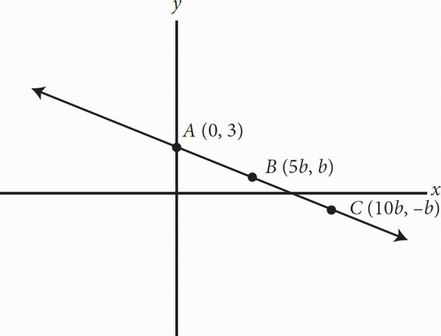The graph of f(x) is shown above in the xy-plane. The points (0, 3), (5b, b), and (10b, -b) are on the line described by f(x). If b is a positive constant, what are the coordinates of point C ?

• A. (5, 1)
• B. (10, -1)
• C. (15, -0.5)
• D. (20, -2)

BRight away, (A) can be eliminated, since point C has a negative y-coordinate. Given any two points, the slope of the line can be determined using the equation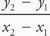. Use this formula to find the value of b by setting the slope of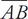equal to the slope of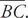Use points (0, 3) and (5b, b) in the left side of the equation and points (5b, b) and (10b, -b) in the right side of the equation to get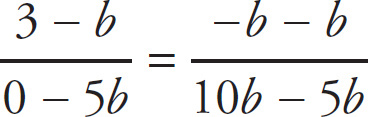. Simplify both sides of the equation to get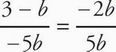, or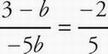. Cross-multiply to get 5(3 - b) = 10b. Divide both sides by 5 get 3 - b = 2b, then 3 = 3b, and finally b = 1. Plug in b =1 for point C to get [10(1), - (1)], or (10, -1). Therefore, the correct answer is (B).